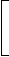# Data Interpretation - Pie Charts

Directions to Solve

Study the following graph and the table and answer the questions given below.

Data of different states regarding population of states in the year 1998Total population of the given States = 3276000.

 States Sex and Literacy wise Population Ratio Sex Literacy M F Literate Illiterate A.P 5 3 2 7 M.P 3 1 1 4 Delhi 2 3 2 1 Goa 3 5 3 2 Bihar 3 4 4 1 U.P. 3 2 7 2 T.N. 3 4 9 4

1.
What will be the percentage of total number of males in U.P., M.P. and Goa together to the total population of all the given states?
25%
27.5%
28.5%
31.5%
Explanation:

 Number of males in U.P =3 of (15% of N)= 3 x 15 x N   = 9 x N . 5 5 100 100

where N = 3276000.

 Number of males in M.P =3 of (20% of N)= 3 x 20 x N   = 15 x N . 4 4 100 100

 Number of males in Goa =3 of (12% of N)= 3 x 12 x N   = 4.5 x N . 8 8 100 100Total number of males in these three states
 = (9 + 15 + 4.5) x N 100
 =28.5 x N. 100Required Percentage =28.5 x N100
x 100% = 28.5%.
N

2.
What was the total number of illiterate people in A.P. and M.P. in 1998?
876040
932170
981550
1161160
Explanation:

 No. of illiterate people in A.P. =7 of (25% of 3276000)= 637000. 9

 No. of illiterate people in M.P. =4 of (20% of 3276000)= 524160. 5Total number = (637000 + 524160) = 1161160.

3.
What is the ratio of the number of females in T.N. to the number of females in Delhi?
7 : 5
9 : 7
13 : 11
15 : 14
Explanation:

Required ratio
=
 4 of (9% of 3276000) 7
 3 of (8% of 3276000) 5
=4 x 973 x 85
 =4 x 9 x 5 x 17 3 8
 = 15 . 14

4.
What was the number of males in U.P. in the year 1998?
254650
294840
321470
341200
Explanation:

Number of males in U.P.
 =3 of (15% of 3276000)5
 = 3 x 15 x 3726000 5 100
= 294840.

5.
If in the year 1998, there was an increase of 10% in the population of U.P. and 12% in the population of M.P. compared to the previous year, then what was the ratio of populations of U.P. and M.P. in 1997?
42 : 55
48 : 55
7 : 11
4 : 5
Explanation:

Let x be the population of U.P. in 1997. Then,

 Population of U.P. in 1998 = 110% of x = 110 x x. 100

Also, let y be the population of M.P. in 1997. Then,

 Population of M.P. in 1998 = 112% of y = 112 x y. 100

Ratio of populations of U.P. and M.P. in 1998 =110 x x100
= 110x .112 x y100
112y

 From the pie-chart, this ratio is 15 . 20110x = 15x = 15 x 112 = 42 . 112y 20 y 20 110 55

Thus, ratio of populations of U.P. and M.P. in 1997 = x : y = 42 : 55.